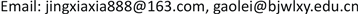﻿ 一类线性乘积和规划问题的分支定界缩减方法 A Branch and Bound Reduction Algorithm for Solving a Class of Sums of Linear Multiplicative Programming Problems

Operations Research and Fuzziology
Vol.07 No.04(2017), Article ID:22415,6 pages
10.12677/ORF.2017.74012

A Branch and Bound Reduction Algorithm for Solving a Class of Sums of Linear Multiplicative Programming Problems

Xia Jing, Lei Gao

School of Mathematics and Information Science, Baoji University of Arts and Sciences, Baoji ShaanxiReceived: Oct. 3rd, 2017; accepted: Oct. 15th, 2017; published: Oct. 23rd, 2017ABSTRACT

In this paper, we consider a class of sums of linear multiplicative programming problems. Using two-stage relaxation technique, a new lower bound of the objective function is obtained. If so, we only solve a sequence of linear programming problems. Moreover, we use a rectangular reduction strategy to provide a new branch and bound reduction algorithm for determining the lower bound of global optimal value. Numerical examples are given to illustrate the obtained results.

Keywords:The Global Value, Linear Multiplicative Programming, Branch and BoundCopyright © 2017 by authors and Hans Publishers Inc.

This work is licensed under the Creative Commons Attribution International License (CC BY).

http://creativecommons.org/licenses/by/4.0/1. 引言

$\left(GLMP\right)\left\{\begin{array}{l}\mathrm{min}\text{}f\left(x\right)=\underset{j=1}{\overset{p}{\sum }}{\delta }_{j}{n}_{j}\left(x\right){d}_{j}\left(x\right)\\ s.t.\text{}x\in D=\left\{x\in {R}^{n}|Ax\le b\right\}\end{array}$

2. 松弛定下界方法

$\left\{\begin{array}{l}\mathrm{min}\text{}{n}_{j}\left(x\right)\\ s.t.\text{}x\in D\cap {H}^{0}\end{array}$$\left\{\begin{array}{l}\mathrm{max}\text{}{n}_{j}\left(x\right)\\ s.t.\text{}x\in D\cap {H}^{0}\end{array}$$\left\{\begin{array}{l}\mathrm{min}\text{}{d}_{j}\left(x\right)\\ s.t.\text{}x\in D\cap {H}^{0}\end{array}$$\left\{\begin{array}{l}\mathrm{max}\text{}{d}_{j}\left(x\right)\\ s.t.\text{}x\in D\cap {H}^{0}\end{array}$ (1)

${\underset{_}{\epsilon }}_{j}\le {n}_{j}\left(x\right)\le {\stackrel{¯}{\epsilon }}_{j}$${\underset{_}{\eta }}_{j}\le {d}_{j}\left(x\right)\le {\stackrel{¯}{\eta }}_{j}$ (2)

${\Omega }_{j}=\left[{\underset{_}{\epsilon }}_{j},{\stackrel{¯}{\epsilon }}_{j}\right]×\left[{\underset{_}{\eta }}_{j},{\stackrel{¯}{\eta }}_{j}\right]$$j=1,2,\cdots ,p$ 。下面确定乘积函数 ${n}_{j}\left(x\right){d}_{j}\left(x\right)$${\Omega }_{j}$ 上的凸包络  ：

1) 若 $\left({n}_{j}-{\underset{_}{\epsilon }}_{j}\right)\ge 0$$\left({d}_{j}-{\underset{_}{\eta }}_{j}\right)\ge 0$ ，则

$\left({n}_{j}-{\underset{_}{\epsilon }}_{j}\right)\left({d}_{j}-{\underset{_}{\eta }}_{j}\right)\ge 0$

${n}_{j}{d}_{j}\ge {\underset{_}{\eta }}_{j}{n}_{j}+{\underset{_}{\epsilon }}_{j}{d}_{j}-{\underset{_}{\epsilon }}_{j}{\underset{_}{\eta }}_{j}$

2) 若 $\left({n}_{j}-{\stackrel{¯}{\epsilon }}_{j}\right)\le 0$$\left({d}_{j}-{\stackrel{¯}{\eta }}_{j}\right)\le 0$ ，则

${n}_{j}{d}_{j}\ge {\stackrel{¯}{\eta }}_{j}{n}_{j}+{\stackrel{¯}{\epsilon }}_{j}{d}_{j}-{\stackrel{¯}{\epsilon }}_{j}{\stackrel{¯}{\eta }}_{j}$

3) 若 $\left({n}_{j}-{\underset{_}{\epsilon }}_{j}\right)\ge 0$$\left({d}_{j}-{\stackrel{¯}{\eta }}_{j}\right)\le 0$ ，则

${n}_{j}{d}_{j}\le {\stackrel{¯}{\eta }}_{j}{n}_{j}+{\underset{_}{\epsilon }}_{j}{d}_{j}-{\underset{_}{\epsilon }}_{j}{\stackrel{¯}{\eta }}_{j}$

4) 若 $\left({n}_{j}-{\stackrel{¯}{\epsilon }}_{j}\right)\le 0$$\left({d}_{j}-{\underset{_}{\eta }}_{j}\right)\ge 0$ ，则

${n}_{j}{d}_{j}\le {\underset{_}{\eta }}_{j}{n}_{j}+{\stackrel{¯}{\epsilon }}_{j}{d}_{j}-{\stackrel{¯}{\epsilon }}_{j}{\underset{_}{\eta }}_{j}$

${\underset{_}{\eta }}_{j}{n}_{j}+{\underset{_}{\epsilon }}_{j}{d}_{j}-{\underset{_}{\epsilon }}_{j}{\underset{_}{\eta }}_{j}\triangleq {\underset{_}{f}}_{\text{ }j}^{1}\left(x\right)$${\stackrel{¯}{\eta }}_{j}{n}_{j}+{\stackrel{¯}{\epsilon }}_{j}{d}_{j}-{\stackrel{¯}{\epsilon }}_{j}{\stackrel{¯}{\eta }}_{j}\triangleq {\underset{_}{f}}_{\text{ }j}^{2}\left(x\right)$

${\stackrel{¯}{\eta }}_{j}{n}_{j}+{\underset{_}{\epsilon }}_{j}{d}_{j}-{\underset{_}{\epsilon }}_{j}{\stackrel{¯}{\eta }}_{j}\triangleq {\stackrel{¯}{f}}_{j}^{1}\left(x\right)$${\underset{_}{\eta }}_{j}{n}_{j}+{\stackrel{¯}{\epsilon }}_{j}{d}_{j}-{\stackrel{¯}{\epsilon }}_{j}{\underset{_}{\eta }}_{j}\triangleq {\stackrel{¯}{f}}_{j}^{2}\left(x\right)$

$\mathrm{max}\left\{{\underset{_}{f}}_{\text{ }j}^{1}\left(x\right),{\underset{_}{f}}_{\text{ }j}^{2}\left(x\right)\right\}\le {n}_{j}{d}_{j}\le \mathrm{min}\left\{{\stackrel{¯}{f}}_{j}^{1}\left(x\right),\text{}{\stackrel{¯}{f}}_{j}^{2}\left(x\right)\right\}$

$\left(SLMP\right)\left\{\begin{array}{l}\text{min}\underset{j=1}{\overset{{p}_{1}}{\sum }}\mathrm{max}\left\{{\underset{_}{f}}_{\text{ }j}^{1}\left(x\right),{\underset{_}{f}}_{\text{ }j}^{2}\left(x\right)\right\}-\underset{j={p}_{1}+1}{\overset{p}{\sum }}\mathrm{min}\left\{{\stackrel{¯}{f}}_{j}^{1}\left(x\right),\text{}{\stackrel{¯}{f}}_{j}^{2}\left(x\right)\right\}\\ s.t.\text{}x\in D\cap {H}^{k}\text{}\end{array}$

$\underset{j=1}{\overset{{p}_{1}}{\sum }}\mathrm{max}\left\{{\underset{_}{f}}_{\text{ }j}^{1}\left(x\right),{\underset{_}{f}}_{\text{ }j}^{2}\left(x\right)\right\}\ge \mathrm{max}\left\{\underset{j=1}{\overset{{p}_{1}}{\sum }}{\underset{_}{f}}_{\text{ }j}^{1}\left(x\right),\underset{j=1}{\overset{{p}_{1}}{\sum }}{\underset{_}{f}}_{\text{ }j}^{2}\left(x\right)\right\}$

$\underset{j={p}_{1}+1}{\overset{p}{\sum }}\mathrm{min}\left\{{\stackrel{¯}{f}}_{j}^{1}\left(x\right),\text{}{\stackrel{¯}{f}}_{j}^{2}\left(x\right)\right\}\le \mathrm{min}\left\{\underset{j={p}_{1}+1}{\overset{p}{\sum }}{\stackrel{¯}{f}}_{j}^{1}\left(x\right),\underset{j={p}_{1}+1}{\overset{p}{\sum }}{\stackrel{¯}{f}}_{j}^{2}\left(x\right)\right\}$

$\left(SSLP\right)\left\{\begin{array}{l}\text{min}\underset{_}{f}\left(x\right)=\mathrm{max}\left\{\underset{j=1}{\overset{{p}_{1}}{\sum }}{\underset{_}{f}}_{\text{ }j}^{1}\left(x\right),\underset{j=1}{\overset{{p}_{1}}{\sum }}{\underset{_}{f}}_{\text{ }j}^{2}\left(x\right)\right\}-\mathrm{min}\left\{\underset{j={p}_{1}+1}{\overset{p}{\sum }}{\stackrel{¯}{f}}_{j}^{1}\left(x\right),\underset{j={p}_{1}+1}{\overset{p}{\sum }}{\stackrel{¯}{f}}_{j}^{2}\left(x\right)\right\}\\ s.t.\text{}x\in D\cap {H}^{k}\end{array}$

(SSLP)问题的最优解为(GLMP)问题的一个可行解，其最优值恰好为(GLMP)问题在超矩形 ${H}^{k}$ 上全局最优值的一个下界，该可行解记为 ${x}^{k}$ ，令 $Q=Q\cup \left\{{x}^{k}\right\}$ ，其中Q表示(GLMP)问题目前所有可行解构成的集合。

$\left(LP\right)\left\{\begin{array}{l}\mathrm{min}\underset{_}{f}\left(x,{t}_{1},{t}_{2}\right)={t}_{1}-{t}_{2}\\ s.t.\text{}\underset{j=1}{\overset{{p}_{1}}{\sum }}{\underset{_}{f}}_{\text{ }j}^{1}\left(x\right)-{t}_{1}\le 0,\\ \text{}\underset{j=1}{\overset{{p}_{1}}{\sum }}{\underset{_}{f}}_{\text{ }j}^{2}\left(x\right)-{t}_{1}\le 0,\\ \text{}\underset{j={p}_{1}+1}{\overset{p}{\sum }}{\stackrel{¯}{f}}_{j}^{1}\left(x\right)-{t}_{2}\ge 0,\\ \text{}\underset{j={p}_{1}+1}{\overset{p}{\sum }}{\stackrel{¯}{f}}_{j}^{2}\left(x\right)-{t}_{2}\ge 0,\text{}\\ \text{}x\in D=\left\{x\in {R}^{n}|Ax\le \text{b}\right\}\cap {H}^{k}=\left[{v}^{k},{h}^{k}\right]\end{array}$

3. 分支定界算法

3.1. 超矩形的剖分

1) 选择 ${H}^{k}$ 的最长边，即 ${h}_{\xi }^{k}-{v}_{\xi }^{k}=\mathrm{max}\left\{{h}_{i}^{k}-{v}_{i}^{k}:i=1,2,\cdots ,n\right\}$

2) 令 ${x}_{\xi }^{k}=\frac{{v}_{\xi }^{k}+{h}_{\xi }^{k}}{2}$$\stackrel{¯}{x}=\left({v}_{1}^{k},{v}_{2}^{k},\cdots ,{v}_{i-1}^{k},{x}_{\xi }^{k},{v}_{i+1}^{k},\cdots ,{v}_{n}^{k}\right)$

${H}_{1}^{k+}{}^{1}=\left[{v}^{k+1,1},{h}^{k+1,1}\right]$ , ${H}_{2}^{k+}{}^{1}=\left[{v}^{k+1,2},{h}^{k+1,2}\right]$

${v}^{k\text{+}1,1}={\left({v}_{1}^{k\text{+}1,1},{v}_{2}^{k\text{+}1,1},\cdots ,{v}_{n}^{k\text{+}1,1}\right)}^{\text{T}}$${h}^{k\text{+}1,1}={\left({h}_{1}^{k\text{+}1,1},\cdots ,{h}_{i-1}^{k\text{+}1,1},{x}_{\xi }^{k},{h}_{i+1}^{k\text{+}1,1},\cdots ,{h}_{n}^{k\text{+}1,1}\right)}^{\text{T}}$

${h}^{k\text{+}1,2}={\left({h}_{1}^{k\text{+}1,2},{h}_{2}^{k\text{+}1,2},\cdots ,{h}_{n}^{k\text{+}1,2}\right)}^{\text{T}}$${v}^{k\text{+}1,2}={\left({v}_{1}^{k\text{+}1,2},\cdots ,{v}_{i-1}^{k\text{+}1,2},{x}_{\xi }^{k},{v}_{i+1}^{k\text{+}1,2},\cdots ,{v}_{n}^{k\text{+}1,2}\right)}^{\text{T}}$

$P=\left\{{H}_{1}^{k+1},{H}_{2}^{k+1}\right\}$$T=\left(T\\left\{{H}^{k}\right\}\right)\cup P$

3.2. 超矩形的缩减

3.3. 分支定界算法

D：(GLMP)问题的可行域；Q：当前可行解的集合； ${H}^{k}$ ：选择剖分的超矩形；

T：剪支剩余超矩形的集合； $\tau$ ：(GLMP)问题全局最优值的上界；

$\eta$ ：(GLMP)问题全局最优值的下界。

$\gamma -\eta \le \sigma$$T=\Phi$ ，则终止，输出(GLMP)问题的全局最优解 ${x}^{*}$ 、全局最优值 $f\left({x}^{*}\right)$ ; 否则，转步骤3；

$T$ 中选择 ${H}^{k}$${H}^{k}$$\eta$ 所对应的超矩形，即 $\eta =\eta \left({H}^{k}\right)$

$k←k+1$ ，转步骤2。

4. 数值实验

$\left\{\begin{array}{l}\mathrm{min}\text{}\left(2{x}_{1}-2{x}_{2}+{x}_{3}+2\right)×\left(-2{x}_{1}+3{x}_{2}+{x}_{3}-4\right)+\left(-2{x}_{1}+{x}_{2}+{x}_{3}+2\right)\\ \text{\hspace{0.17em}}\text{\hspace{0.17em}}\text{\hspace{0.17em}}\text{\hspace{0.17em}}\text{\hspace{0.17em}}\text{\hspace{0.17em}}\text{\hspace{0.17em}}\text{\hspace{0.17em}}×\left({x}_{1}+{x}_{2}-3{x}_{3}+5\right)+\left(-2{x}_{1}-{x}_{2}+2{x}_{3}+7\right)×\left(4{x}_{1}-{x}_{2}-2{x}_{3}-5\right)\\ s.t.\text{}{x}_{1}+{x}_{2}+{x}_{3}\le 10\\ \text{}\text{ }{x}_{1}-2{x}_{2}+3{x}_{3}\le 10\\ \text{}-2{x}_{1}+2{x}_{2}+3{x}_{3}\le 10\\ \text{}-{x}_{1}+2{x}_{2}-3{x}_{3}\le 10\\ \text{}-{x}_{1}+2{x}_{2}+3{x}_{3}\ge 6\\ \text{}{x}_{1}\ge 1,{x}_{2}\ge 1,\text{}{x}_{3}\ge 1\end{array}$

A Branch and Bound Reduction Algorithm for Solving a Class of Sums of Linear Multiplicative Programming Problems[J]. 运筹与模糊学, 2017, 07(04): 104-109. http://dx.doi.org/10.12677/ORF.2017.74012

1. 1. Maranas, C.D., Androulakis, I.P., Floudas, C.A., et al. (1997) Solving Long-Term Financial Planning Problems via Global Optimization. Journal of Economic Dynamics and Control, 21, 1405-1425. https://doi.org/10.1016/S0165-1889(97)00032-8

2. 2. Mulvey, J.M., Vanderbei, R.J. and Zenios, S.A. (1995) Robust Optimization of Large-Scale Systems. Operations Research, 43, 264-281. https://doi.org/10.1287/opre.43.2.264

3. 3. Konno, H. and Watanabe, H. (1996) Bond Portfolio Optimization Problems and Their Application to Index Tracking: A Partial Optimization Approach. Journal of the Operations Research Society of Japan, 39, 295-306. https://doi.org/10.15807/jorsj.39.295

4. 4. Kuno, T, Yajima, Y. and Konno, H. (1993) An Outer Approximation Method for Minimizing the Product of Several Convex Functions on a Convex Set. Journal of Global optimization, 3, 325-335. https://doi.org/10.1007/BF01096774

5. 5. 高岳林, 邓光智. 凹二次规划问题的一个融合割平面方法的分支定界混合算法[J]. 工程数学学报, 2008(25): 548-596.

6. 6. 高岳林, 井霞. 一类线性乘积规划问题的分支定界缩减方法[J]. 计算数学, 2013(35): 89-98.

7. 7. Zhou, X.-G., Cao, B.-Y. and Wu, K. (2015) Global Minimization Method for Linear Multiplicative Programming. Acta. Mathematical Application Sinica, 31, 325-334. https://doi.org/10.1007/s10255-015-0456-6

8. 8. 黄红选, 译. 全局优化引论[M]. 北京: 清华大学出版社, 2003, 1-43.

9. 9. Ryoo, H.S. and Sahinidis, N.V. (2003) Global Optimization of Multiplicative Programs. Journal of Global Optimization, 26, 387-418. https://doi.org/10.1023/A:1024700901538